### 2 多层Transformer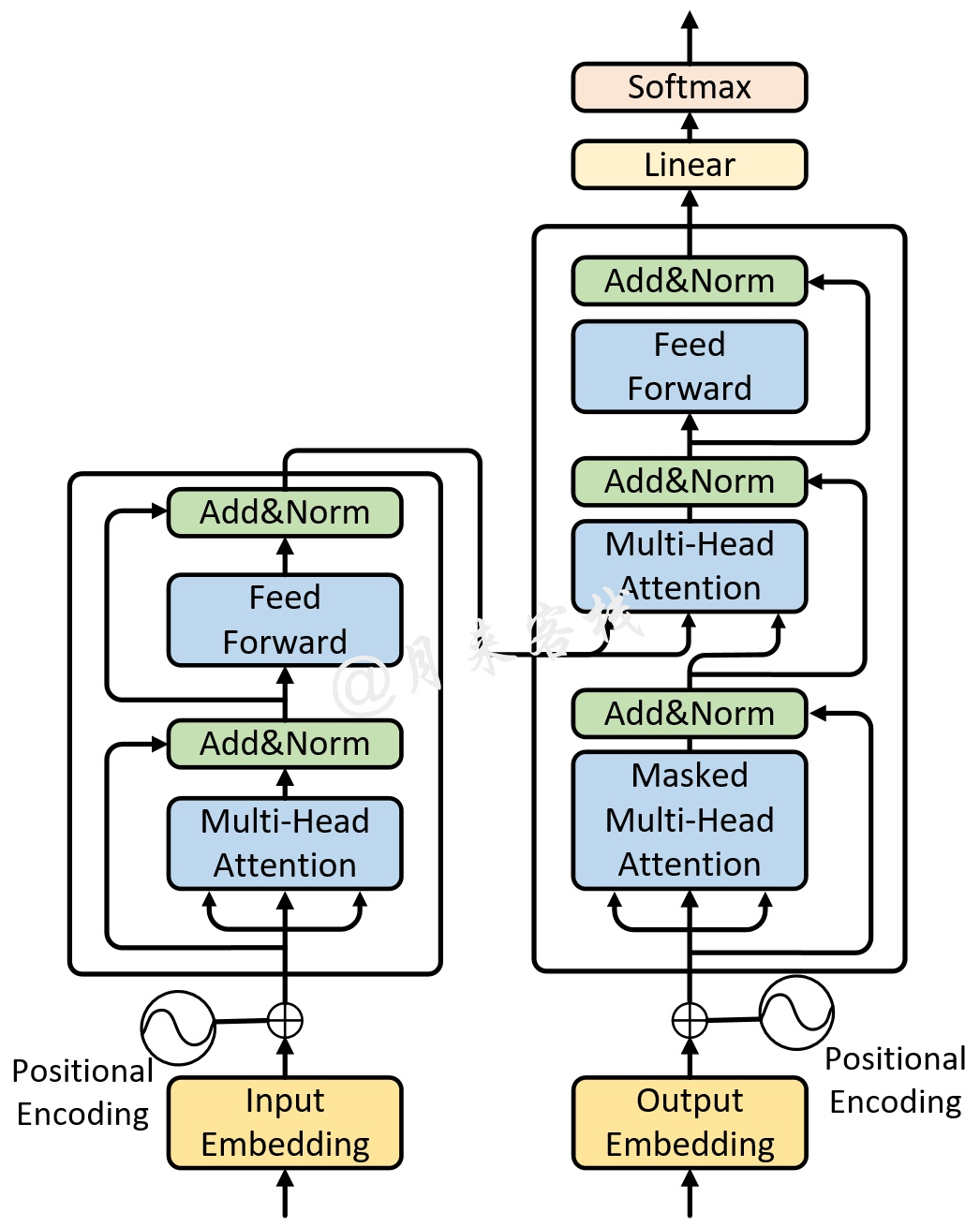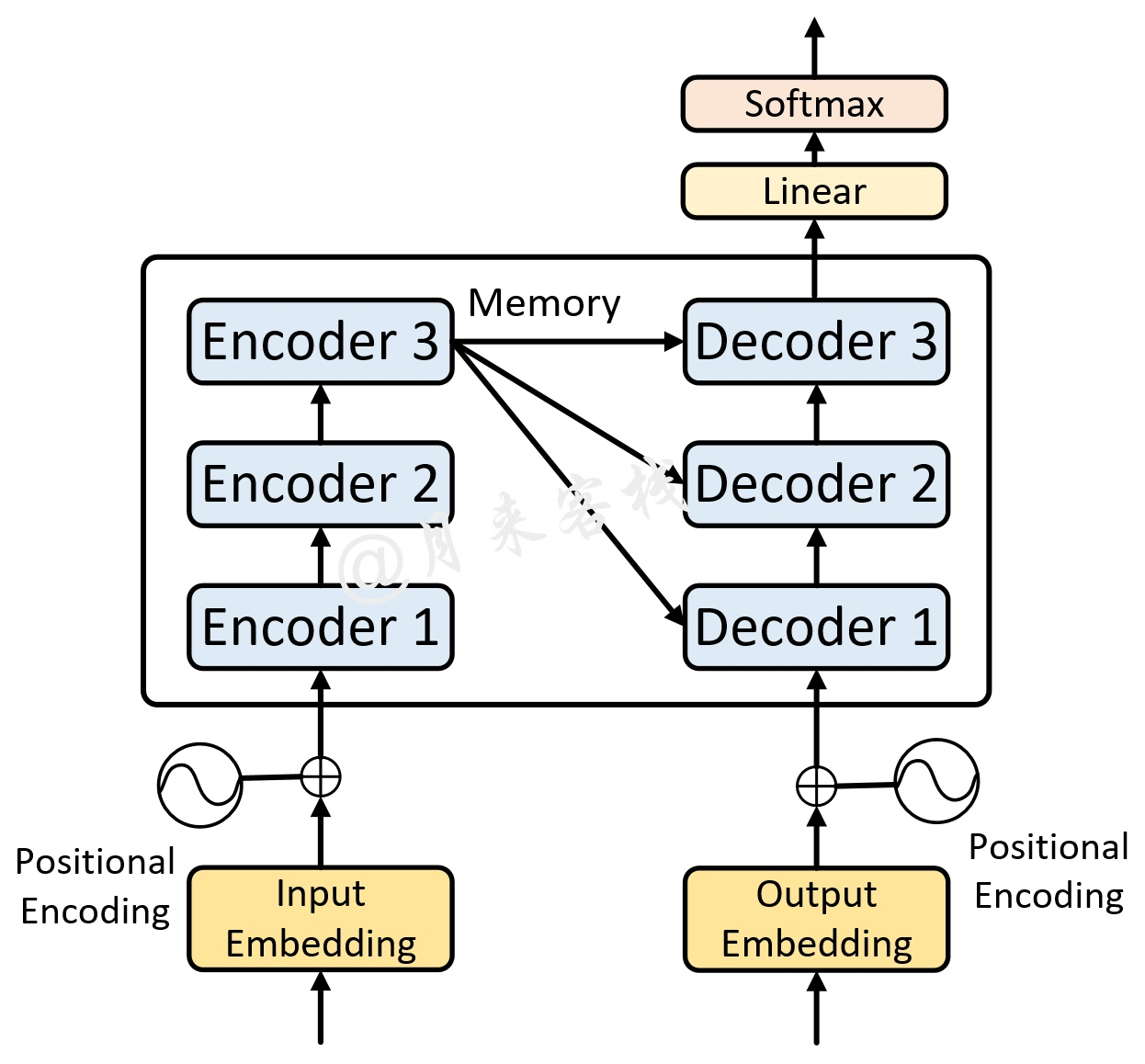### 3 Transformer中的掩码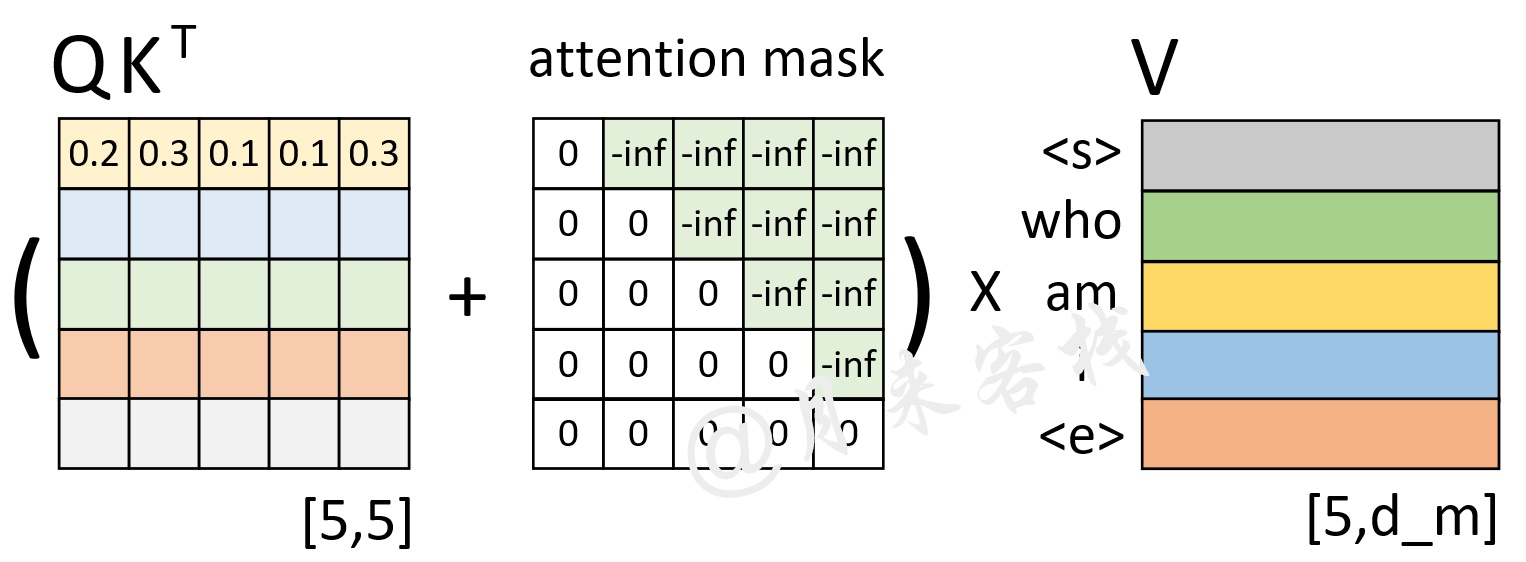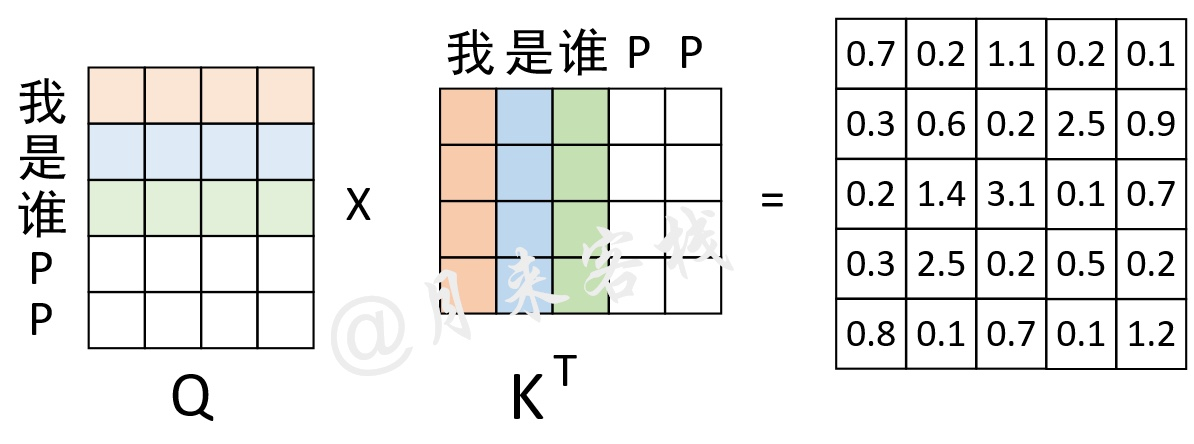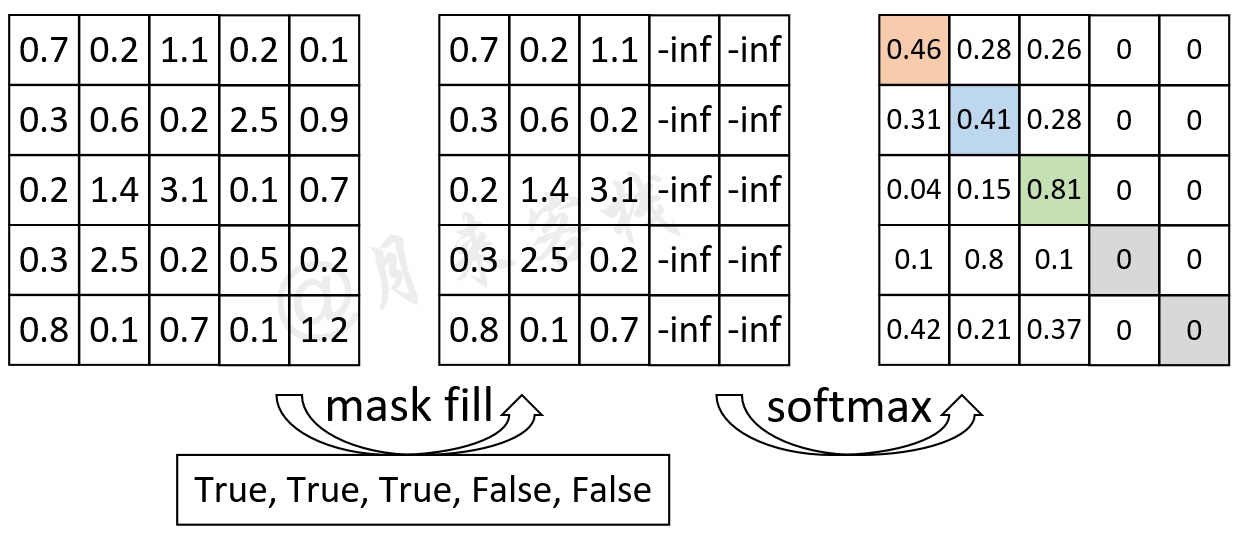### 4 实现多头注意力机制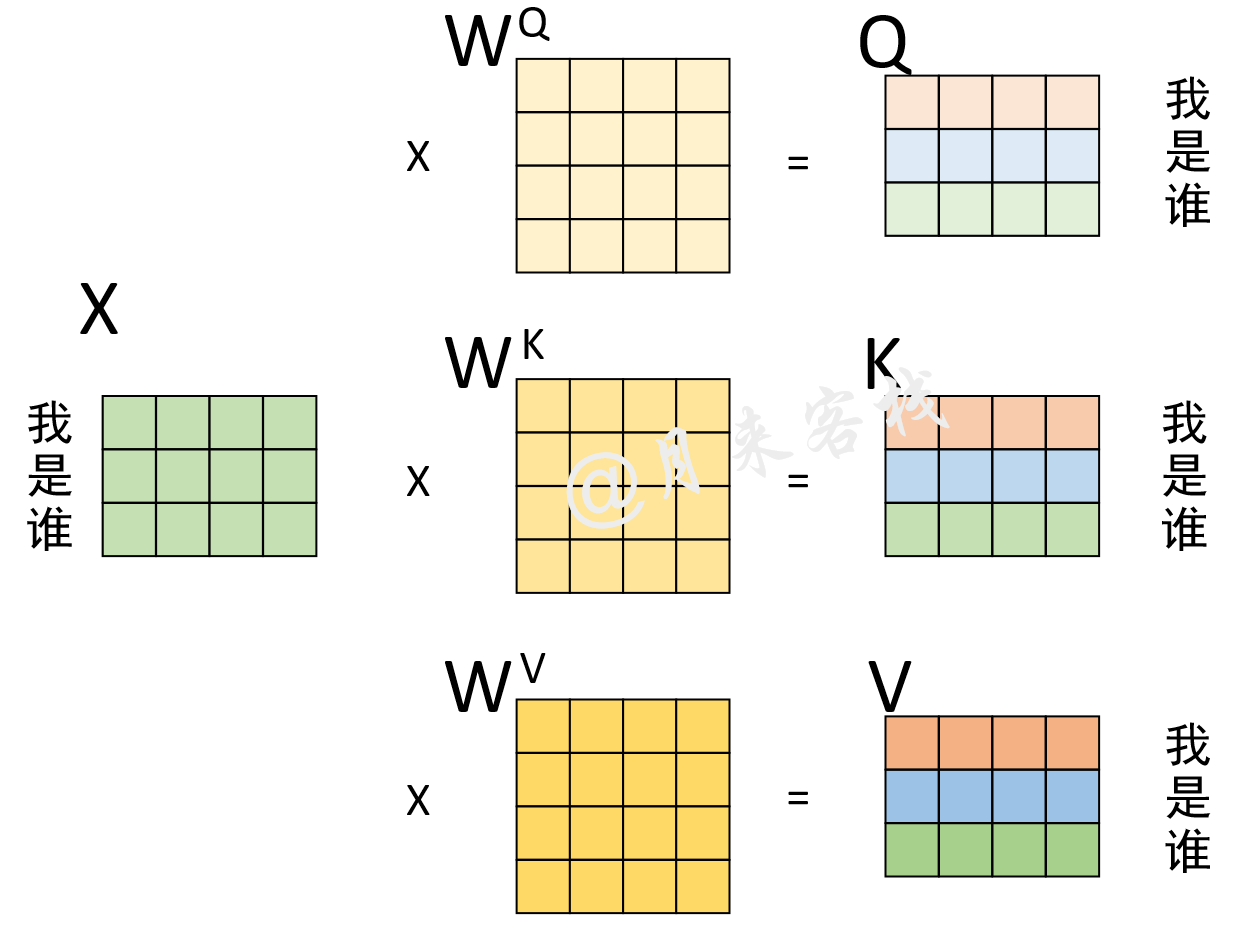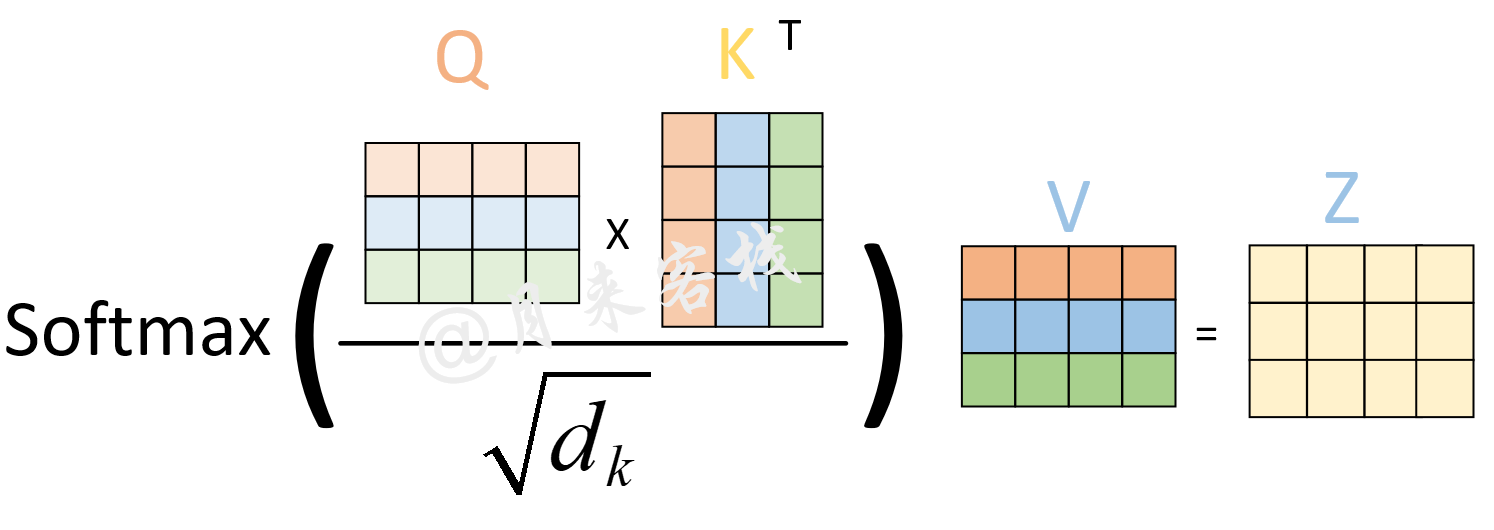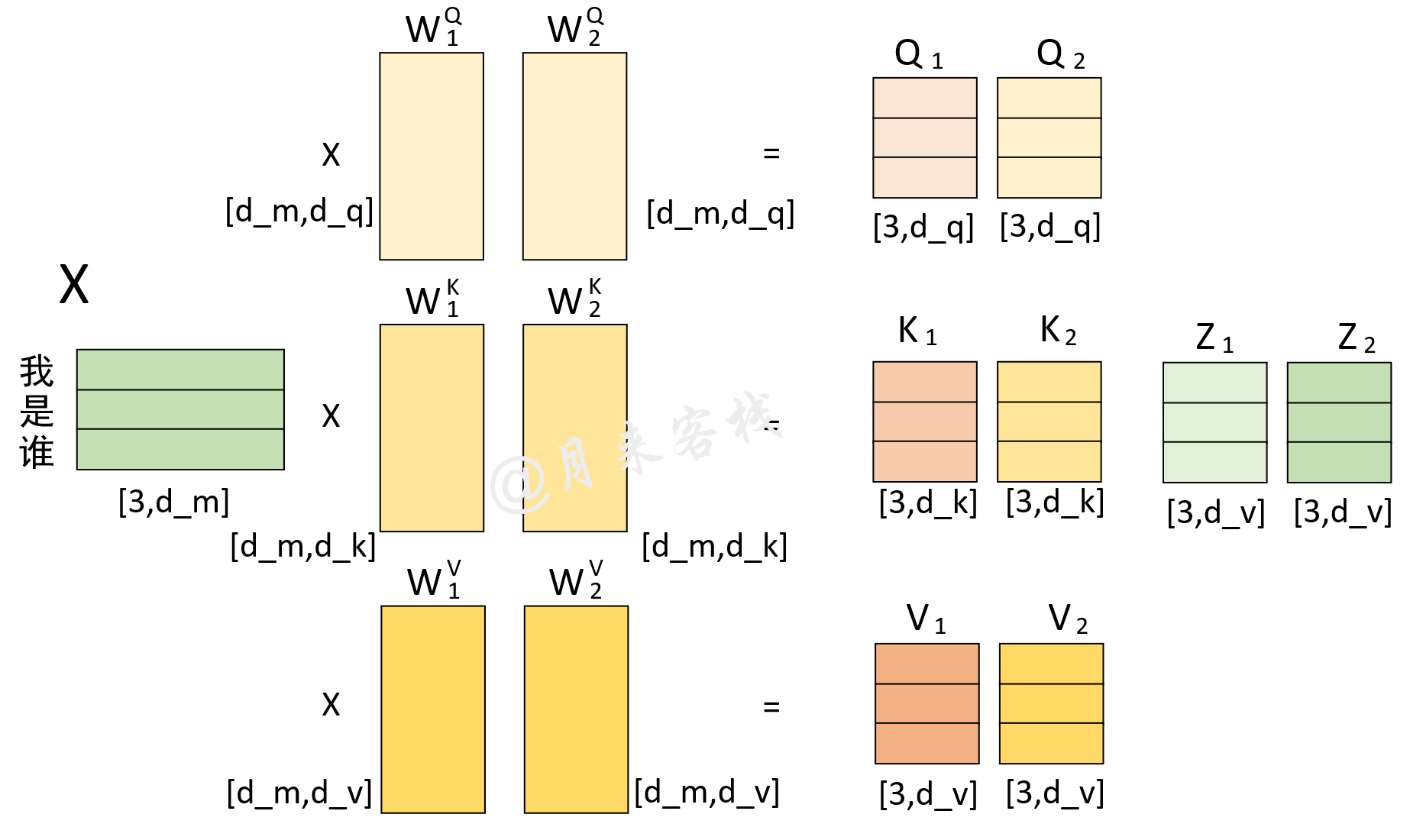#### 4.1 定义类MyMultiHeadAttention

1class MyMultiheadAttention(nn.Module):2    def __init__(self, embed_dim, num_heads, dropout=0., bias=True):3        super(MyMultiheadAttention, self).__init__()4        """5        :param embed_dim:   词嵌入的维度，也就是前面的d_model参数，论文中的默认值为5126        :param num_heads:   多头注意力机制中多头的数量，也就是前面的nhead参数， 论文默认值为 87        :param bias:        最后对多头的注意力（组合）输出进行线性变换时，是否使用偏置8        """9        self.embed_dim = embed_dim  # 前面的d_model参数10        self.head_dim = embed_dim // num_heads  # head_dim 指的就是d_k,d_v11        self.kdim = self.head_dim12        self.vdim = self.head_dim13        self.num_heads = num_heads  # 多头个数14        self.dropout = dropout15        assert self.head_dim * num_heads == self.embed_dim, "embed_dim 除以 num_heads必须为整数"16        # 上面的限制条件就是论文中的  d_k = d_v = d_model/n_head 条件17        self.q_proj_weight = Parameter(torch.Tensor(embed_dim, embed_dim))  # embed_dim = kdim * num_heads18        # 这里第二个维度之所以是embed_dim，实际上这里是同时初始化了num_heads个W_q堆叠起来的, 也就是num_heads个头19        self.k_proj_weight = Parameter(torch.Tensor(embed_dim, embed_dim))  # W_k,  embed_dim = kdim * num_heads20        self.v_proj_weight = Parameter(torch.Tensor(embed_dim, embed_dim))  # W_v,  embed_dim = vdim * num_heads21        self.out_proj = nn.Linear(embed_dim, embed_dim, bias=bias)22        # 最后将所有的Z组合起来的时候，也是一次性完成， embed_dim = vdim * num_heads

#### 4.2 定义前向传播过程

xxxxxxxxxx231    def forward(self, query, key, value, attn_mask=None, key_padding_mask=None):2        """3        在论文中，编码时query, key, value 都是同一个输入， 4        解码时 输入的部分也都是同一个输入， 5        解码和编码交互时 key,value指的是 memory, query指的是tgt6        :param query: # [tgt_len, batch_size, embed_dim], tgt_len 表示目标序列的长度7        :param key:  #  [src_len, batch_size, embed_dim], src_len 表示源序列的长度8        :param value: # [src_len, batch_size, embed_dim], src_len 表示源序列的长度9        :param attn_mask: # [tgt_len,src_len] or [num_heads*batch_size,tgt_len, src_len]10                一般只在解码时使用，为了并行一次喂入所有解码部分的输入，所以要用mask来进行掩盖当前时刻之后的位置信息11        :param key_padding_mask: [batch_size, src_len], src_len 表示源序列的长度12        :return:13        attn_output: [tgt_len, batch_size, embed_dim]14        attn_output_weights: # [batch_size, tgt_len, src_len]15        """16        return multi_head_attention_forward(query, key, value, self.num_heads,17                                            self.dropout, self.out_proj.weight, self.out_proj.bias,18                                            training=self.training,19                                            key_padding_mask=key_padding_mask,20                                            q_proj_weight=self.q_proj_weight,21                                            k_proj_weight=self.k_proj_weight,22                                            v_proj_weight=self.v_proj_weight,23                                            attn_mask=attn_mask)

#### 4.3 多头注意力计算过程

x1def multi_head_attention_forward(2    query,  # [tgt_len,batch_size, embed_dim]3    key,  # [src_len, batch_size, embed_dim]4    value,  # [src_len, batch_size, embed_dim]5    num_heads,6    dropout_p,7    out_proj_weight, # [embed_dim = vdim * num_heads, embed_dim]8    out_proj_bias,9    training=True,10    key_padding_mask=None,  # [batch_size,src_len/tgt_len]11    q_proj_weight=None,  # [embed_dim,kdim * num_heads]12    k_proj_weight=None,  # [embed_dim, kdim * num_heads]13    v_proj_weight=None,  # [embed_dim, vdim * num_heads]14    attn_mask=None,  # [tgt_len,src_len] 15  ):16    # 第一阶段： 计算得到Q、K、V17    q = F.linear(query, q_proj_weight)18    #  [tgt_len,batch_size,embed_dim] x [embed_dim,kdim * num_heads] = [tgt_len,batch_size,kdim * num_heads]19    k = F.linear(key, k_proj_weight)20    # [src_len, batch_size,embed_dim] x [embed_dim,kdim * num_heads] = [src_len,batch_size,kdim * num_heads]21    v = F.linear(value, v_proj_weight)22    # [src_len, batch_size,embed_dim] x [embed_dim,vdim * num_heads] = [src_len,batch_size,vdim * num_heads]

​x1    # 第二阶段： 缩放，以及attn_mask维度判断2       tgt_len, bsz, embed_dim = query.size()  # [tgt_len,batch_size, embed_dim]3    src_len = key.size(0)4    head_dim = embed_dim // num_heads  # num_heads * head_dim = embed_dim5    scaling = float(head_dim) ** -0.56    q = q * scaling  # [query_len,batch_size,kdim * num_heads]7​8    if attn_mask is not None:  # [tgt_len,src_len] or [num_heads*batch_size,tgt_len, src_len]9        if attn_mask.dim() == 2:10            attn_mask = attn_mask.unsqueeze(0)  # [1, tgt_len,src_len]11            if list(attn_mask.size()) != [1, query.size(0), key.size(0)]:12                raise RuntimeError('The size of the 2D attn_mask is not correct.')13        elif attn_mask.dim() == 3:14            if list(attn_mask.size()) != [bsz * num_heads, query.size(0), key.size(0)]:15                raise RuntimeError('The size of the 3D attn_mask is not correct.')16        # 现在 atten_mask 的维度就变成了3D

xxxxxxxxxx91       # 第三阶段： 计算得到注意力权重矩阵2       q = q.contiguous().view(tgt_len, bsz * num_heads, head_dim).transpose(0, 1)3    # [batch_size * num_heads,tgt_len,kdim]4    # 因为前面是num_heads个头一起参与的计算，所以这里要进行一下变形，以便于后面计算。 且同时交换了0，1两个维度5    k = k.contiguous().view(-1, bsz*num_heads, head_dim).transpose(0,1)#[batch_size * num_heads,src_len,kdim]6    v = v.contiguous().view(-1, bsz*num_heads, head_dim).transpose(0,1)#[batch_size * num_heads,src_len,vdim]7    attn_output_weights = torch.bmm(q, k.transpose(1, 2))8    # [batch_size * num_heads,tgt_len,kdim] x [batch_size * num_heads, kdim, src_len]9    # =  [batch_size * num_heads, tgt_len, src_len]  这就num_heads个QK相乘后的注意力矩阵

xxxxxxxxxx111    # 第四阶段： 进行相关掩码操作2    if attn_mask is not None:3        attn_output_weights += attn_mask  # [batch_size * num_heads, tgt_len, src_len]4    if key_padding_mask is not None:5        attn_output_weights = attn_output_weights.view(bsz, num_heads, tgt_len, src_len)6        # 变成 [batch_size, num_heads, tgt_len, src_len]的形状7        attn_output_weights = attn_output_weights.masked_fill(8            key_padding_mask.unsqueeze(1).unsqueeze(2), float('-inf')) 9         # 扩展维度，从[batch_size,src_len]变成[batch_size,1,1,src_len]10        attn_output_weights = attn_output_weights.view(bsz * num_heads, tgt_len,src_len)  11        # [batch_size * num_heads, tgt_len, src_len]

xxxxxxxxxx151       attn_output_weights = F.softmax(attn_output_weights, dim=-1)# [batch_size * num_heads, tgt_len, src_len]2    attn_output_weights = F.dropout(attn_output_weights, p=dropout_p, training=training)3    attn_output = torch.bmm(attn_output_weights, v)4    # Z = [batch_size * num_heads, tgt_len, src_len]  x  [batch_size * num_heads,src_len,vdim]5    # = # [batch_size * num_heads,tgt_len,vdim]6    # 这就num_heads个Attention(Q,K,V)结果7​8    attn_output = attn_output.transpose(0, 1).contiguous().view(tgt_len, bsz, embed_dim)9    # 先transpose成 [tgt_len, batch_size* num_heads ,kdim]10    # 再view成 [tgt_len,batch_size,num_heads*kdim]11    attn_output_weights = attn_output_weights.view(bsz, num_heads, tgt_len, src_len)12​13    Z = F.linear(attn_output, out_proj_weight, out_proj_bias)14    # 这里就是多个z  线性组合成Z  [tgt_len,batch_size,embed_dim]15    return Z, attn_output_weights.sum(dim=1) / num_heads  # 将num_heads个注意力权重矩阵按对应维度取平均

#### 4.4 示例代码

xxxxxxxxxx111if __name__ == '__main__':2    src_len = 53    batch_size = 24    dmodel = 325    num_head = 16    src = torch.rand((src_len, batch_size, dmodel))  # shape: [src_len, batch_size, embed_dim]7    src_key_padding_mask = torch.tensor([[True, True, True, False, False],8                                         [True, True, True, True, False]])  # shape: [src_len, src_len]9​10    my_mh = MyMultiheadAttention(embed_dim=dmodel, num_heads=num_head)11    r = my_mh(src, src, src,key_padding_mask = src_key_padding_mask)

xxxxxxxxxx181进入多头注意力计算:2   多头num_heads = 1, d_model=32, d_k = d_v = d_model/num_heads=323   query的shape([tgt_len, batch_size, embed_dim]):torch.Size([5, 2, 32])4    W_q 的shape([embed_dim,kdim * num_heads]):torch.Size([32, 32])5     Q  的shape([tgt_len, batch_size,kdim * num_heads]):torch.Size([5, 2, 32])6  ----------------------------------------------------------------------7    key 的shape([src_len,batch_size, embed_dim]):torch.Size([5, 2, 32])8    W_k 的shape([embed_dim,kdim * num_heads]):torch.Size([32, 32])9     K  的shape([src_len,batch_size,kdim * num_heads]):torch.Size([5, 2, 32])10  ----------------------------------------------------------------------11   value的shape([src_len,batch_size, embed_dim]):torch.Size([5, 2, 32])12    W_v 的shape([embed_dim,vdim * num_heads]):torch.Size([32, 32])13     V  的shape([src_len,batch_size,vdim * num_heads]):torch.Size([5, 2, 32])14  ----------------------------------------------------------------------15   ***** 注意，这里的W_q, W_k, W_v是多个head同时进行计算的. 因此，Q,K,V分别也是包含了多个head的q,k,v堆叠起来的结果 *****16   多头注意力中,多头计算结束后的形状（堆叠）为([tgt_len,batch_size,num_heads*kdim])torch.Size([5, 2, 32])17   多头计算结束后，再进行线性变换时的权重W_o的形状为([num_heads*vdim, num_heads*vdim  ])torch.Size([32, 32])18   多头线性变化后的形状为([tgt_len,batch_size,embed_dim]) torch.Size([5, 2, 32])

### 推荐阅读

 This post is all you need（②位置编码与编码解码过程）

 This post is all you need（①多头注意力机制原理）